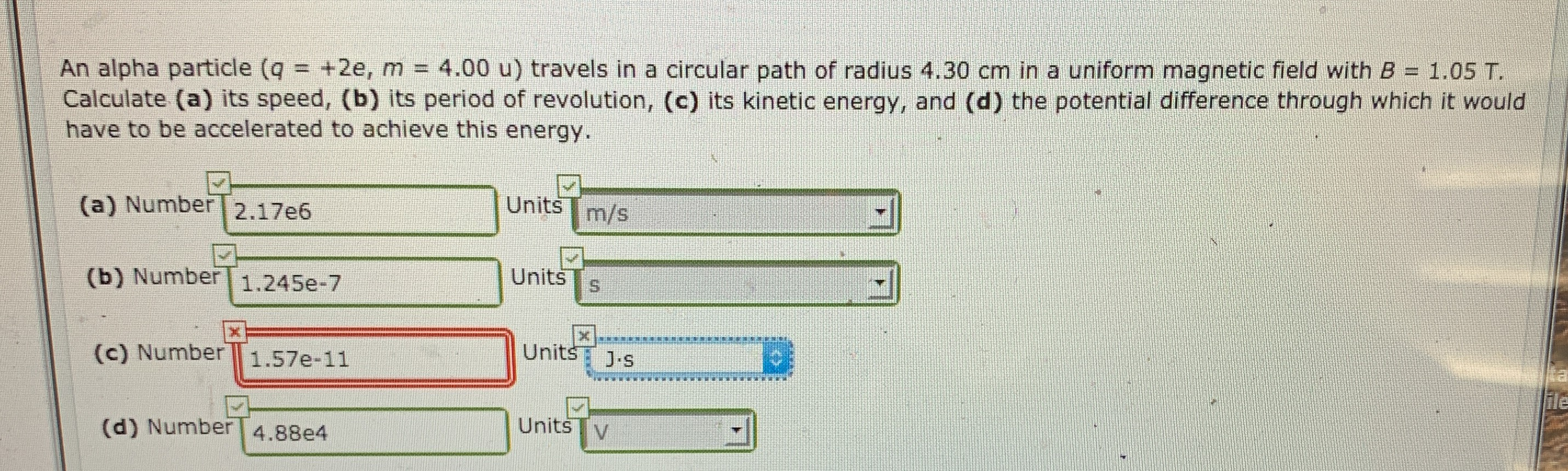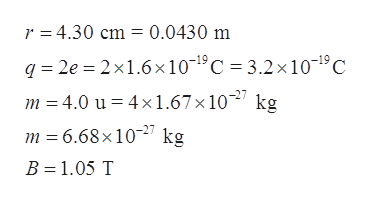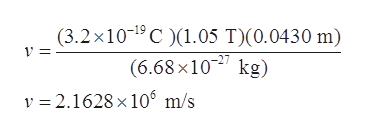# An alpha particle (q = +2e, m=4.00 u) travels in a circular path of radius 4.30 cm in a uniform magnetic field with B 1.05 T.Calculate (a) its speed, (b) its period of revolution, (c) its kinetic energy, and (d) the potential difference through which it wouldhave to be accelerated to achieve this energy.Units(a) Number2.17e6m/sUnits(b) Number1.245e-7XXUnits(c) Number1.57e-11J.sile(d) Number 4.88e4UnitsV

Question
204 viewshelp_outlineImage TranscriptioncloseAn alpha particle (q = +2e, m=4.00 u) travels in a circular path of radius 4.30 cm in a uniform magnetic field with B 1.05 T. Calculate (a) its speed, (b) its period of revolution, (c) its kinetic energy, and (d) the potential difference through which it would have to be accelerated to achieve this energy. Units (a) Number 2.17e6 m/s Units (b) Number 1.245e-7 X X Units (c) Number 1.57e-11 J.s ile (d) Number 4.88e4 Units V fullscreen
check_circle

Step 1

Since we only answer up to 3 sub-parts, we’ll answer the first 3. Please resubmit the question and specify the other subparts (up to 3) you’d like answered.

Given,

The radius of circular path r with charge of alpha particle q and mass of alpha particle m and magnetic field B.help_outlineImage Transcriptioncloser 4.30 cm 0.0430 m q2e 2x1.6x10-1C 3.2 x 10-19C m 4.0 u 4x1.67x 1027 kg m 6.68x 1027 kg B 1.05 T fullscreen
Step 2

Part(a)

Here, the magnetic force (Fmag) contributes in centripetal force (Fcentripetal) i.e.:

Step 3

Now, plugging the given...help_outlineImage Transcriptionclose(3.2x1019 C 1.05 T)(0.0430 m) (6.68 x 10 kg) v 2.1628 x 106 m/s fullscreen

### Want to see the full answer?

See Solution

#### Want to see this answer and more?

Solutions are written by subject experts who are available 24/7. Questions are typically answered within 1 hour.*

See Solution
*Response times may vary by subject and question.
Tagged in

### Physics